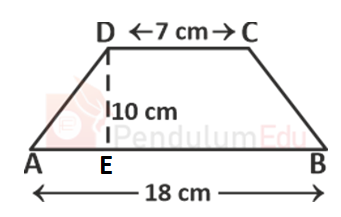Question of The Day21-02-2022

ABCD is a trapezium where AB || CD, AB = 18 cm and CD = 7 cm. Distance between the parallel sides is 10 cm. find the area of trapezium

Correct Answer : a ) 125 cm2

Explanation :

According to the question

AB = 18 cm and CD = 7 cm

AB || CDWe know that

Area of Trapezium=$$1\over2$$(Sum of parallel sides)*height

Substituting the values

Area of trapezium=$$1 \over 2$$(18+7)*10

⇒ 125cm2

Hence, (a) is the correct answer.

Read about various topics of Maths, Science, General Awareness and English in the Study Material section provided by the PendulumEdu.

1. Coordinate Geometry formula and Tricks

2. Time and Work Tricks

3. Percentage Concepts and Tricks0